# Smooth scheme

(diff) ← Older revision | Latest revision (diff) | Newer revision → (diff)

A generalization of the concept of a non-singular algebraic variety. A schemeof (locally) finite type over a fieldis called a smooth scheme (over) if the scheme obtained fromby replacing the field of constantswith its algebraic closure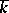is a regular scheme, i.e. if all its local rings are regular. For a perfect fieldthe concepts of a smooth scheme overand a regular scheme overare identical. In particular, a smooth scheme of finite type over an algebraically closed field is a non-singular algebraic variety. In the case of the field of complex numbers a non-singular algebraic variety has the structure of a complex analytic manifold.

A scheme is smooth if and only if it can be covered by smooth neighbourhoods. A point of a schemeis called a simple point of the scheme if in a certain neighbourhood of itis smooth; otherwise the point is called a singular point. A connected smooth scheme is irreducible. A product of smooth schemes is itself a smooth scheme. In general, ifis a smooth scheme overandis a smooth morphism, thenis a smooth scheme over.

An affine space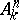and a projective spaceare smooth schemes over; any algebraic group (i.e. a reduced algebraic group scheme) over a perfect field is a smooth scheme. A reduced scheme over an algebraically closed field is smooth in an everywhere-dense open set.

If a schemeis defined by the equations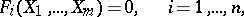in an affine space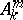, then a pointis simple if and only if the rank of the Jacobi matrix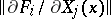is equal to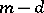, whereis the dimension ofat(Jacobi's criterion). In a more general case, a closed subschemeof a smooth schemedefined by a sheaf of idealsis smooth in a neighbourhood of a pointif and only if there exists a system of generators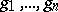of the ideal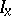in the ring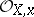for whichform part of a basis of a free-module of the differential sheaf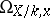.

How to Cite This Entry:
Smooth scheme. Encyclopedia of Mathematics. URL: http://encyclopediaofmath.org/index.php?title=Smooth_scheme&oldid=11424
This article was adapted from an original article by V.I. DanilovI.V. Dolgachev (originator), which appeared in Encyclopedia of Mathematics - ISBN 1402006098. See original article